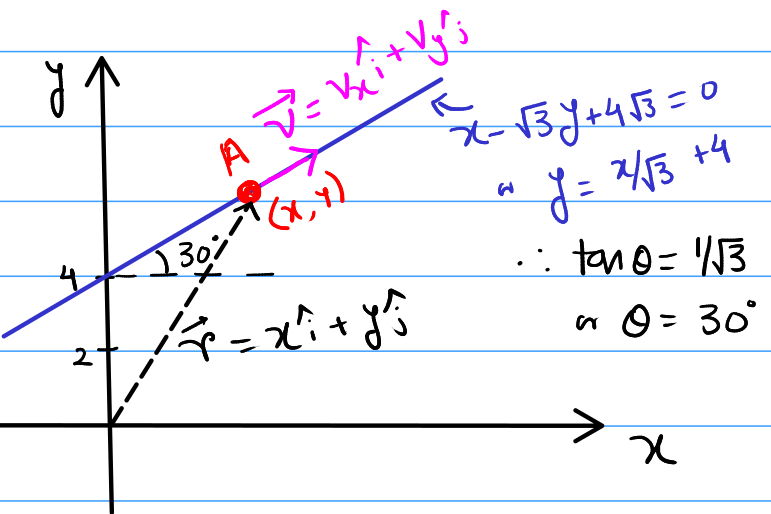How Cheenta works to ensure student success?
Explore the Back-Story

# NSEP 2015 Problem 3 | Rotational MotionTry out this problem on the concept of Angular momentum from National Standard Examination in Physics 2015-2016.

## NSEP 2015-16 ~ Problem 3

A particle of mass 10g starts from rest at $t=0$s from point (0m, 4m) and gets accelerated at $0.5m/ s^2$ along $x-\sqrt{3}y + 4\sqrt{3}=0$ in XY plane. The angular momentum of the particle about the origin (in SI units) at $t=2$s is
(a) $-0.01\sqrt{3} \hat{k}$
(b) $-0.02\sqrt{3}\hat{k}$
(c) Zero
(d) $-20\sqrt{3}\hat{k}$

### Key Concepts

Circular Motion

Center of Curvature for generalised motion

Vector and their Components

## Suggested Book | Source | Answer

Concept of Physics H.C. Verma

University Physics by H. D. Young and R.A. Freedman

Fundamental of Physics D. Halliday, J. Walker and R. Resnick

National Standard Examination in Physics(NSEP) 2015-2016

Option-(b) $-0.02\sqrt{3}\hat{k}$

## Try with Hints

The first thing to remember is that Even if a particle is not moving in a circle, then also it can have circular motion, i.e., Angular Momentum, Torque, Centripetal forces can exist.

This is the case because any random curves can be approximated bu circles. Even straight lines are circles with radius $\to \infty$.

You can use the circle's centre as the centre of rotation or you can also use the origin. Using the origin is often easier as the positions can be directly given using position vectors.

In our problem, The motion is happening on the line $y = \frac{x}{\sqrt{3}}+4$.

If at any moment of time it's velocity along the curve is $\vec{v}=v_x \hat{i}+v_y \hat{j}$ and it's position vector is $\vec{r}=x \hat{i}+y \hat{j}$ then, It's angular momentum ($\vec{L}$) with respect to origin can be given by,

$$\vec{L} = m(\vec{v}\times \vec{r})$$

where m is the mass of the particle.

From the previous image, if $(x,y)$ is then they must satisfy $y = \frac{x}{\sqrt{3}}+4$. Differentiating with respect to time,

$$\frac{dy}{dt} = \frac{1}{\sqrt{3}}\frac{dx}{dt} \to v_y = \frac{v_x}{\sqrt{3}}$$

Again differentiating w.r.t time,

$$a_y = \frac{a_x}{\sqrt{3}}$$ where $\vec{a} = a_x\hat{i}+a_y\hat{j}$ is the acceleration (we will not need this but I think it should be written here as we can also use this in solution).

Now at $t=0$, $v_x=0$. Hence, at $t=2s$, $v_x$=$a_xt$= $a\cos(30^{\circ})t$= $0.5\frac{\sqrt{3}}{2} 2$= $\frac{\sqrt{3}}{2}$

Now, $x$ = $v_x(t=0 )t+\frac{1}{2}a_x t^2$ = $0 + \frac{1}{2}a\cos(30^{\circ})2^2$ = $\frac{\sqrt{3}}{2}$

Then, $v_y = \frac{v_x}{\sqrt{3}}= \frac{1}{2}$ and $y = \frac{x}{\sqrt{3}} + 4 = \frac{1}{2} + 4 = \frac{9}{2}$.

Hence, $\vec{r}$ = $\frac{\sqrt{3}}{2}\hat{i} + \frac{9}{2}\hat{j}$ and $\vec{v}$ = $\frac{\sqrt{3}}{2}\hat{i} + \frac{1}{2}\hat{j}$.

$\vec{L}$=$m(\vec{r} \times \vec{v})$=$-m 2 \sqrt{3} \hat{k}$=$-\left(\frac{10}{1000}\right) 2 \sqrt{3} \hat{k}$=$-0.02 \sqrt{3} \hat{k}$

Note: The answer is asked in S.I unit. So, m = 10g = $\frac{10}{1000}$kg = 0.01.

## Subscribe to Cheenta at Youtube

Try out this problem on the concept of Angular momentum from National Standard Examination in Physics 2015-2016.

## NSEP 2015-16 ~ Problem 3

A particle of mass 10g starts from rest at $t=0$s from point (0m, 4m) and gets accelerated at $0.5m/ s^2$ along $x-\sqrt{3}y + 4\sqrt{3}=0$ in XY plane. The angular momentum of the particle about the origin (in SI units) at $t=2$s is
(a) $-0.01\sqrt{3} \hat{k}$
(b) $-0.02\sqrt{3}\hat{k}$
(c) Zero
(d) $-20\sqrt{3}\hat{k}$

### Key Concepts

Circular Motion

Center of Curvature for generalised motion

Vector and their Components

## Suggested Book | Source | Answer

Concept of Physics H.C. Verma

University Physics by H. D. Young and R.A. Freedman

Fundamental of Physics D. Halliday, J. Walker and R. Resnick

National Standard Examination in Physics(NSEP) 2015-2016

Option-(b) $-0.02\sqrt{3}\hat{k}$

## Try with Hints

The first thing to remember is that Even if a particle is not moving in a circle, then also it can have circular motion, i.e., Angular Momentum, Torque, Centripetal forces can exist.

This is the case because any random curves can be approximated bu circles. Even straight lines are circles with radius $\to \infty$.

You can use the circle's centre as the centre of rotation or you can also use the origin. Using the origin is often easier as the positions can be directly given using position vectors.

In our problem, The motion is happening on the line $y = \frac{x}{\sqrt{3}}+4$.

If at any moment of time it's velocity along the curve is $\vec{v}=v_x \hat{i}+v_y \hat{j}$ and it's position vector is $\vec{r}=x \hat{i}+y \hat{j}$ then, It's angular momentum ($\vec{L}$) with respect to origin can be given by,

$$\vec{L} = m(\vec{v}\times \vec{r})$$

where m is the mass of the particle.

From the previous image, if $(x,y)$ is then they must satisfy $y = \frac{x}{\sqrt{3}}+4$. Differentiating with respect to time,

$$\frac{dy}{dt} = \frac{1}{\sqrt{3}}\frac{dx}{dt} \to v_y = \frac{v_x}{\sqrt{3}}$$

Again differentiating w.r.t time,

$$a_y = \frac{a_x}{\sqrt{3}}$$ where $\vec{a} = a_x\hat{i}+a_y\hat{j}$ is the acceleration (we will not need this but I think it should be written here as we can also use this in solution).

Now at $t=0$, $v_x=0$. Hence, at $t=2s$, $v_x$=$a_xt$= $a\cos(30^{\circ})t$= $0.5\frac{\sqrt{3}}{2} 2$= $\frac{\sqrt{3}}{2}$

Now, $x$ = $v_x(t=0 )t+\frac{1}{2}a_x t^2$ = $0 + \frac{1}{2}a\cos(30^{\circ})2^2$ = $\frac{\sqrt{3}}{2}$

Then, $v_y = \frac{v_x}{\sqrt{3}}= \frac{1}{2}$ and $y = \frac{x}{\sqrt{3}} + 4 = \frac{1}{2} + 4 = \frac{9}{2}$.

Hence, $\vec{r}$ = $\frac{\sqrt{3}}{2}\hat{i} + \frac{9}{2}\hat{j}$ and $\vec{v}$ = $\frac{\sqrt{3}}{2}\hat{i} + \frac{1}{2}\hat{j}$.

$\vec{L}$=$m(\vec{r} \times \vec{v})$=$-m 2 \sqrt{3} \hat{k}$=$-\left(\frac{10}{1000}\right) 2 \sqrt{3} \hat{k}$=$-0.02 \sqrt{3} \hat{k}$

Note: The answer is asked in S.I unit. So, m = 10g = $\frac{10}{1000}$kg = 0.01.

## Subscribe to Cheenta at Youtube

This site uses Akismet to reduce spam. Learn how your comment data is processed.

### Knowledge Partner